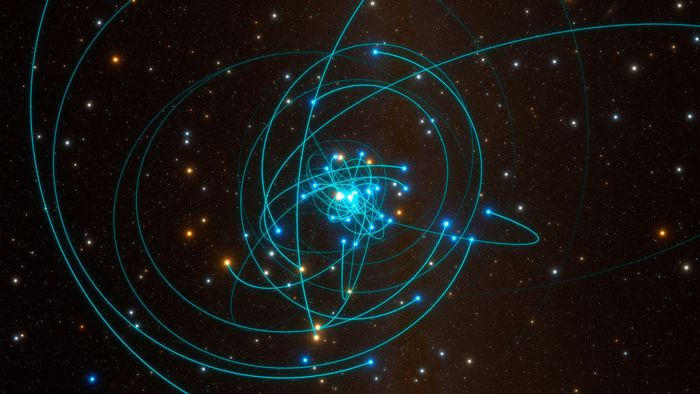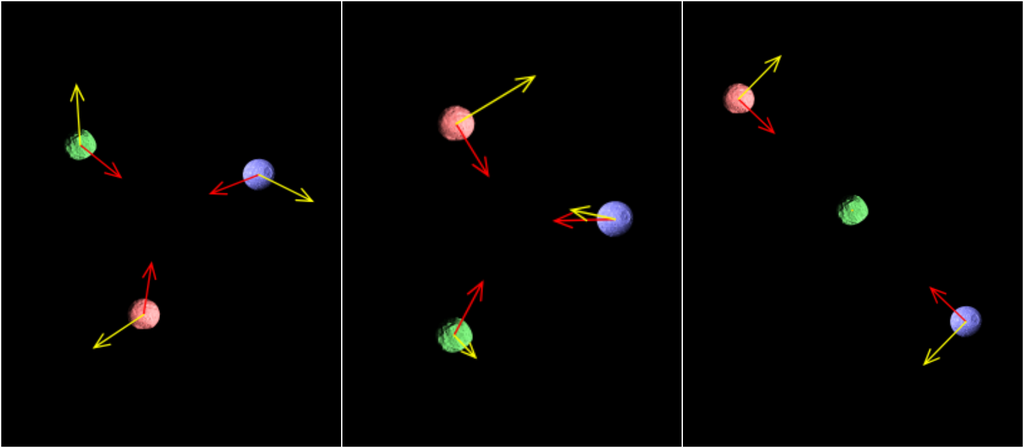Connect with us

Hi, what are you looking for?Are star orbits in complex systems like a drunk's gait?The orbits of systems formed by three celestial bodies can be predicted by a mathematical theory called "random walk", also known as "the drunk walk". According to a new study, the unpredictability of these systems can be used to obtain more accurate calculations.

• Could three nearby black holes collide? Yes, but it depends. Understand!
• How do astronomers calculate the orbits of planets, moons and stars?

The three-body orbit problem

Predicting the orbit of a planet, asteroid, comet or even stars revolving around galactic centers is relatively simple. These bodies are always orbiting around some other object, like the Earth orbits the Sun and the Moon orbits the Earth. The same goes for two-star binary systems.

However, things get complicated when there are three objects orbiting each other — or more. Such systems are fairly easily found in our own galaxy, such as globular clusters with hundreds of stars gravitationally bound together.

A Market Analysis on Youtube: news, product reviews, tips, event coverage and much more! Subscribe to our YouTube channel , every day there's a new video for you!

The difficulty of predicting these orbits has troubled physicists since the days of Isaac Newton, because three objects orbiting each other do not follow a predictable path defined by mathematical formulas. Currently, scientists use computer models and simulations to deal with the problem .

According to the math, if the three objects are of comparable size and distance from the central point, a “struggle” for prevalence takes place, throwing the system into chaos. Therefore, it is impossible to use the classical formulas to predict the orbits, although some less orthodox solutions have already obtained some results.

Stars “walk” like drunks?Simulation of three examples of possible interactions between three bodies shows the multiple possibilities (Image: Reproduction/Javalab/Creative Commons)

The new study proposes to make unpredictability play in math's favor. According to the random walk theory, it is possible to predict a chaotic path if the possibilities are considered.

Using a drunk walking in random directions as an example, there is an equal chance that he will take a step to the right and to the left. If we know all the possibilities, it is possible to calculate the probability that the drunk will end up in a certain place at some time.

In this approach, three-body systems, where the third object approaches a pair of objects in mutual orbit, have velocity "steps" during the approach. "One can calculate what the probabilities are for each of these possible third-body velocities," said Yonadav Barry Ginat, a doctoral candidate at the Israel Technion Institute of Technology.

According to Ginat, “you can compose all these steps and all these probabilities to find the final probability of what is going to happen to the three-body system after a long time.” This means that physicists could know if the third object will be ejected from the surface. system, for example, or an elliptical orbit will form, to get closer again.

The paper, published in the journal Physical Review X, solves even more complex problems than the speed of approaching the third body. For example, the different and complex interactions between stars and planets — like the Moon's gravitational forces pulling the Earth to produce the tides.

In other solutions, these complexities are often ignored because they add even more hurdles to solving the problem. Tidal forces steal some energy from the interaction between two bodies, for example, and this changes the way each object moves, adding more chaos to the system.

But with the proposal to use random walk math, this can be circumvented, as it calculates the probability of each "step" of the three-body interaction. Thus, it is possible to solve how these additional forces interfere in the system and calculate the result more accurately.

The study authors admit that this solution is not definitive, although it is a big step. They now hope to find out what happens when the three bodies are in special configurations and whether it is possible to use the same approach for four-body systems.

Read the article on A Market Analysis .

Trending on A Market Analysis:

• Deltacron: Another variant of the coronavirus is discovered in Cyprus
• charm | 6 Easter Eggs You Probably Haven't Seen
• Redmi 10 vs Galaxy A32 comparison: which is better
• Samsung Galaxy S22: Announcement, pre-order and launch dates leak
• Ransomware uses popular remote management program to infect machines